﻿

Blood donors targeting using machine learning

# Target donors in blood donation campaigns using artificial intelligence

This study aims to predict if a person will donate blood by using a recency, frequency, monetary, and time (RFMT) marketing model.

We took the database used for this study from the donor database of Blood Transfusion Service Center in Hsin-Chu City in Taiwan.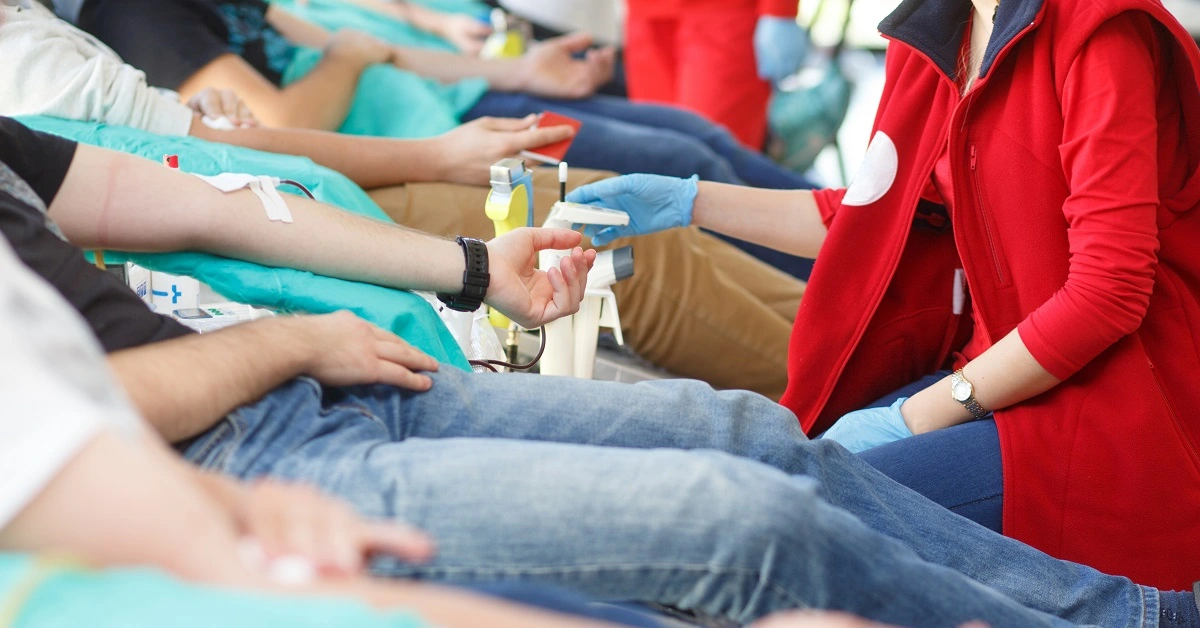### Contents:

This example is solved with Neural Designer. You can use free trial to follow it step by step.

## 1. Application type

The variable to be predicted is binary (donate or not). Therefore, this is a binary classification project,

We aim to model the probability of a person donating blood, conditioned on their features, using artificial intelligence and machine learning.

## 2. Data set

The data file blood_donation.csv contains the information used to create the model. It consists of 748 rows and five columns. The columns represent the variables, and the rows represent the instances.

The number of input variables, or attributes for each sample, is 5. All input variables are numeric-valued and represent features from blood donors. The target variable is donation, being 0 no blood donation and 1 blood donation for the last campaign. The following list summarizes the variables information:

The next list describes the variables information:

• recency: Months since the last donation.
• frequency: Total number of donations.
• quantity: Total blood donated.
• time: Months since the first donation.
• donation: True if the person donated in the last campaign, false otherwise.

Finally, the use of all instances is selected. Each patient has an instance that contains the input and target variables. Neural Designer divides the data into three subsets: training, validation, and testing, automatically assigning 60%, 20%, and 20% of the instances for training, generalization, and testing, respectively. The user can modify these values.

Then we can perform a few related data analyses and check that the data has good quality.

We can calculate the data statistics and draw a table with descriptive statistics (minimums, maximums, means, and standard deviations) of all variables in the data set. The next table depicts the values.

Minimum Maximum Mean Deviation
recency 0 74 9.51 8.1
frequency 1 50 5.51 5.84
quantity 250 1.25e+4 1.38e+3 1.46e+3
time 2 98 34.3 24.4
donation 0 1 0.238 0.426

Also, we can calculate the data distributions for each variable. The following pie chart shows the numbers of donation (positives) and no donation (negatives) donors in the data set.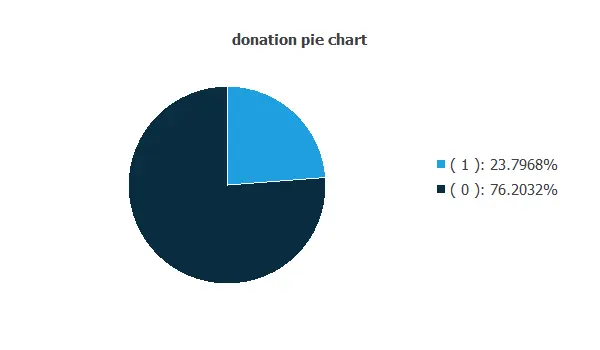As depicted on the image, the number of negative responses, i.e., no donations, is much higher than the number of positive responses, 76%, and 23%, respectively.

The inputs-targets correlations might indicate which factors most influence whether a person would donate blood or not and therefore be more relevant to our analysis.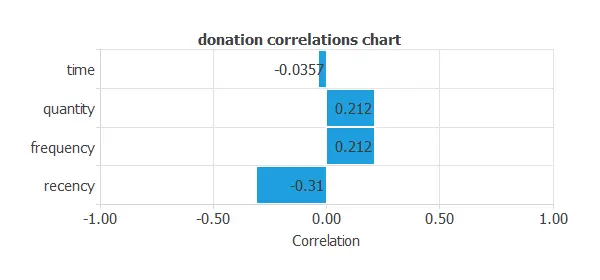Here, the most correlated variables with blood donation are recency, frequency, and quantity. Also if we calculate the correlations between the inputs, quantity and frequency have a correlation of 1. So one can be unused, in this case we will not use quantity as it has a higher magnitude order.

## 3. Neural network

The next step is to set a neural network to represent the classification function. For this type of application, the neural network is composed of:

The scaling layer contains the statistics of the inputs calculated from the data file and the method for scaling the input variables. Here the mean and standard deviation scaling method has been set; this scales the inputs to have mean 0 and standard deviation 1. We usually apply this method to variables with a normal (or Gaussian) distribution.

A perceptron layer with a Hyperbolic tangent activation function The neural network needs four inputs since the number of scaling neurons is four. As a starting point, we use three neurons in the hidden layer.

The probabilistic layer contains the method for interpreting the outputs as probabilities. The output of the output layer’s activation function is logistic and interpretable as our target variable’s probability. This probabilistic layer has three inputs, the same as input variables. Its output represents the probability of a person donating blood, conditioned on their features.

The following figure represents the neural network for blood donor prediction.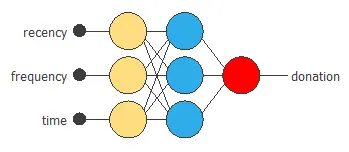## 4. Training strategy

The fourth step is to set the training strategy, which is composed of two terms:

• Loss index.
• An Optimization algorithm.

The loss index chosen is the weighted squared error with L2 regularization.

The learning problem is finding a neural network that minimizes the loss index, or a neural network that fits the data set (error term) and does not oscillate (regularization term).

The optimization algorithm that we use is the quasi-Newton method. This is the standard optimization algorithm for this type of problem.

The following chart shows how the error decreases with the iterations during the training process. The final training and selection errors are training error = 0.778266 WSE and selection error = 0.734308 WSE, respectively.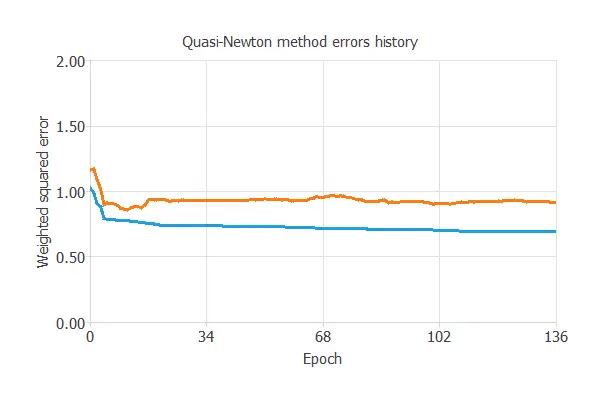## 5. Model selection

The objective of model selection is to find the network architecture that minimizes the error on the selected instances of the data set.

We aim to find a neural network with a selection error lower than 0.734308 WSE, which is the value that we have achieved so far.

Order selection algorithms aim to reduce the selection error training several network architectures with different number of neurons.

The incremental order method increases the number of neurons and their complexity with each iteration. The following graph shows the training error (blue) and selection error (orange) as a function of the number of neurons.

In this case, when we perform a model selection, we slightly improve it, but the model complexity increases too much. Therefore, we opt for maintaining our first model as the final model for our study.## 6. Testing analysis

The objective of the testing analysis is to validate the performance of the trained neural network. To validate a classification technique, we need to compare the values provided by this technique to the observed values. We can use the ROC curve as it is the standard testing method for binary classification projects.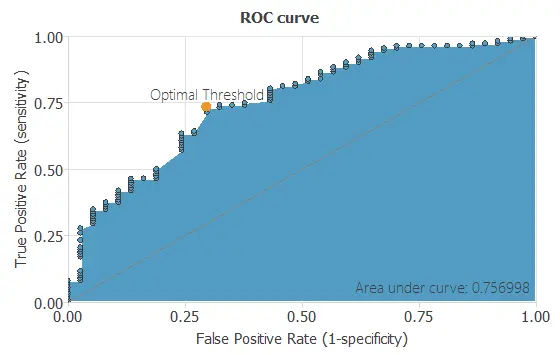A random classifier has an area under a curve of 0.5. in comparison, the perfect classifier would have an area under a curve of 1. In practice, this measure should take a value between 0.5 and 1. The closer to 1, the better the classifier. In this example, this parameter is AUC = 0.804, which means a great performance.

The following table contains the elements of the confusion matrix. This matrix contains the true positives, false positives, false negatives, and true negatives for the variable diagnosis.

Predicted negative Predicted positive
Real negative 72 40
Real positive 10 27

The binary classification tests are parameters for measuring the performance of a classification problem with two classes:

• Classification accuracy: 66.4% (ratio of correctly classified samples).
• Error rate: 33.6% (ratio of misclassified samples).
• Sensitivity: 64.2% (percentage of actual positive classified as positive).
• Specificity: 73% (percentage of actual negative classified as negative).

## 7. Model deployment

Once the generalization performance of the neural network has been tested, it can be saved for future use in the so-called model deployment mode.

We can predict whether a person is going to donate blood by calculating the neural network outputs. For that, we need to set the input variables.

• recency: 9 months since the last donation.
• frequency: 5 number of donations.
• time: 34 months since the first donation.

The predicted donation probability for these values is the following:

• donation: 51% probability.

The objective of the Response Optimization algorithm is to exploit the mathematical model to look for optimal operating conditions.
Indeed, the predictive model allows us to simulate different operating scenarios and adjust the control variables to improve efficiency.

An example is to maximize donation probability while maintaining recency between two desired values and remaining inputs below health limits.

The next table resumes the conditions for this problem.

Variable name Condition
Recency Between 4 12
Frequency Less than 10
Quantity Less than 2000
Time Greater than 4
Donation probability Maximize

The next list shows the optimum values for previous conditions.

• recency: 5 months since the last donation.
• frequency: 9 number of donations.
• frequency: 1582 total donated blood.
• time: 5 months since the first donation.
• donation: 83% probability.

The mathematical expression represented by the neural network is written below. It takes the inputs recency, frequency, monetary, and time to produce the output prediction about donation.

            scaled_recency = (recency-9.506679535)/8.095399857;
scaled_frequency = (frequency-5.514709949)/5.839310169;
scaled_time = (time-34.28210068)/24.37669945;

perceptron_layer_1_output_0 = tanh( 0.358944 + (scaled_recency*-0.692014) + (scaled_frequency*-1.37401) + (scaled_time*-0.531336) );
perceptron_layer_1_output_1 = tanh( 0.675304 + (scaled_recency*0.579182) + (scaled_frequency*1.97605) + (scaled_time*-0.334593) );
perceptron_layer_1_output_2 = tanh( -0.501794 + (scaled_recency*-0.801198) + (scaled_frequency*0.234288) + (scaled_time*-0.228785) );

probabilistic_layer_combinations_0 = -0.27896 +0.832439*perceptron_layer_1_output_0 +1.53477*perceptron_layer_1_output_1 +1.72943*perceptron_layer_1_output_2

donation = 1.0/(1.0 + exp(-probabilistic_layer_combinations_0);


The above expression can be exported anywhere.

## References:

• The data for this problem has been taken from the UCI Machine Learning Repository.
• Yeh, I-Cheng, Yang, King-Jang, and Ting, Tao-Ming, “Knowledge discovery on RFM model using Bernoulli sequence, “Expert Systems with Applications, 2008,
• https://dl.acm.org/citation.cfm?id=1498365